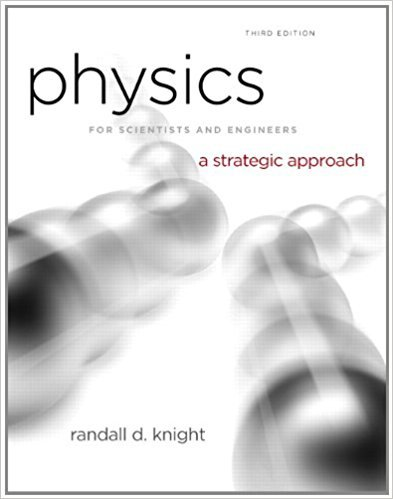×
Log in to StudySoup
Get Full Access to Physics For Scientists And Engineers: A Strategic Approach With Modern Physics - 3 Edition - Chapter 8 - Problem 37p
Join StudySoup for FREE
Get Full Access to Physics For Scientists And Engineers: A Strategic Approach With Modern Physics - 3 Edition - Chapter 8 - Problem 37p

Already have an account? Login here
×
Reset your password

# Two wires are tied to the 2.0 kg sphere shown in FIGURE.ISBN: 9780321740908 69

## Solution for problem 37P Chapter 8

Physics for Scientists and Engineers: A Strategic Approach with Modern Physics | 3rd Edition

• Textbook Solutions
• 2901 Step-by-step solutions solved by professors and subject experts
• Get 24/7 help from StudySoup virtual teaching assistantsPhysics for Scientists and Engineers: A Strategic Approach with Modern Physics | 3rd Edition

4 5 1 328 Reviews
24
2
Problem 37P

Problem 37P

Two wires are tied to the 2.0 kg sphere shown in FIGURE. The sphere revolves in a horizontal circle at constant speed.

a. For what speed is the tension the same in both wires?

b. What is the tension?

FIGUREStep-by-Step Solution:

Solution 37 P:

Step 1 of 2:-

a)

Here we have to find the speed of rotation.

The pictorial representation is given below.Now, let’s break the problem componentwise.

For x direction,---------------------(1)

For y direction,--------------------(2)

Let’s calculateand.

Both are equal to 1.36.

As, the two tensionsandmust be same as per our demand, we can equate equation (1) and (2).-----------------(3)

The isoscale property of the triangle states that,So,.

So, the velocity will be 2.91 m/s.

Step 2 of 2

##### ISBN: 9780321740908

The answer to “Two wires are tied to the 2.0 kg sphere shown in FIGURE. The sphere revolves in a horizontal circle at constant speed.a. For what speed is the tension the same in both wires?________________b. What is the tension?FIGURE” is broken down into a number of easy to follow steps, and 37 words. Physics for Scientists and Engineers: A Strategic Approach with Modern Physics was written by and is associated to the ISBN: 9780321740908. The full step-by-step solution to problem: 37P from chapter: 8 was answered by , our top Physics solution expert on 08/30/17, 04:34AM. Since the solution to 37P from 8 chapter was answered, more than 7437 students have viewed the full step-by-step answer. This textbook survival guide was created for the textbook: Physics for Scientists and Engineers: A Strategic Approach with Modern Physics, edition: 3. This full solution covers the following key subjects: wires, tension, sphere, figure, speed. This expansive textbook survival guide covers 17 chapters, and 1439 solutions.

Unlock Textbook Solution

Enter your email below to unlock your verified solution to:

Two wires are tied to the 2.0 kg sphere shown in FIGURE.